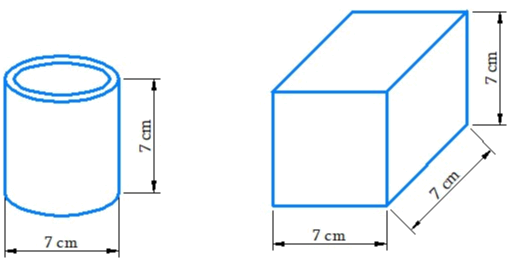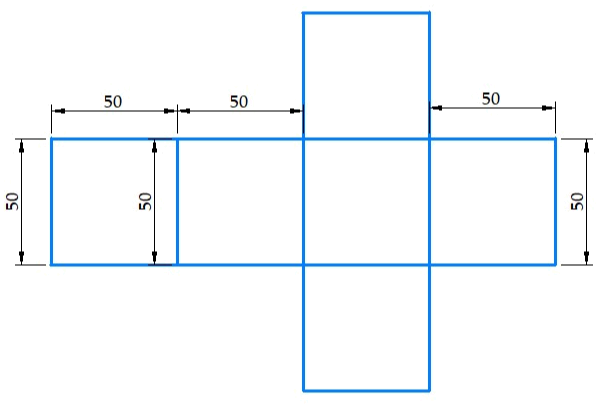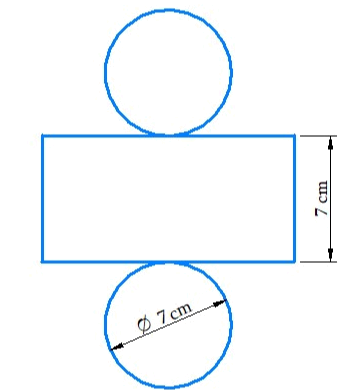# Ex.11.3 Q6 Mensuration Solution - NCERT Maths Class 8

Go back to  'Ex.11.3'

## Question

Describe how the two figures at the right are alike and how they are different. Which box has larger lateral surface area?Video Solution
Mensuration
Ex 11.3 | Question 6

## Text Solution

What is Known?

Shape and their respective dimension.

What is unknown?

Lateral surface area.

Reasoning:Length of rectangular strip will be equal to the circumference of the circle. Visually, all the faces of a cube and square are equal in shape. This makes length, height and width of a cube equal, so area of each of the face will be equal.

Steps:

Similarly, both the figures are alike in respect of their same height.

The difference between the two figures is that one is cylinder and other is a cube.

Length of one side of cube $$\,(l) = 7\, \rm{cm}$$

Height of one side of cube $$\,(h) = 7\, \rm{cm}$$

Width of one side of cube $$\,(b) = 7\, \rm{cm}$$

Lateral surface area of the cube

\begin{align}&= (h\! \times\! l \!+\! h\! \times\! b\!+\! h\! \times \!l \!+\! h\! \times\! b)\\ &= (l\! \times\! l \!+\! l \!\times\! l\! +\! l \times l \!+ \! l \times l)\\&\qquad \because \{ l \!= \!h \!=\! b\} \\&= 4{l^2}\\ &= 4 \times {(7)^2}\\&= 196\,{{\rm{m}}^2} \end{align}

Height of the cylinder $$h = 7\,\rm{cm}$$

\begin{align}r = \frac{7}{2}\,\rm{cm} = 3.5\,\rm{cm} \end{align}

Lateral surface area of the cylinder

\begin{align}&= 2\pi rh\\&= 2 \times \frac{{22}}{7} \times 3.5 \times 7\\&=154\, \rm{m^2} \end{align}

Hence, the cube has larger lateral surface area.

Learn from the best math teachers and top your exams

• Live one on one classroom and doubt clearing
• Practice worksheets in and after class for conceptual clarity
• Personalized curriculum to keep up with school